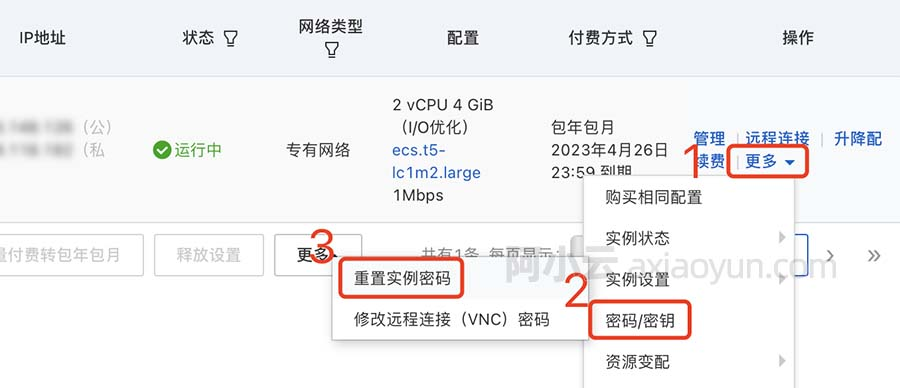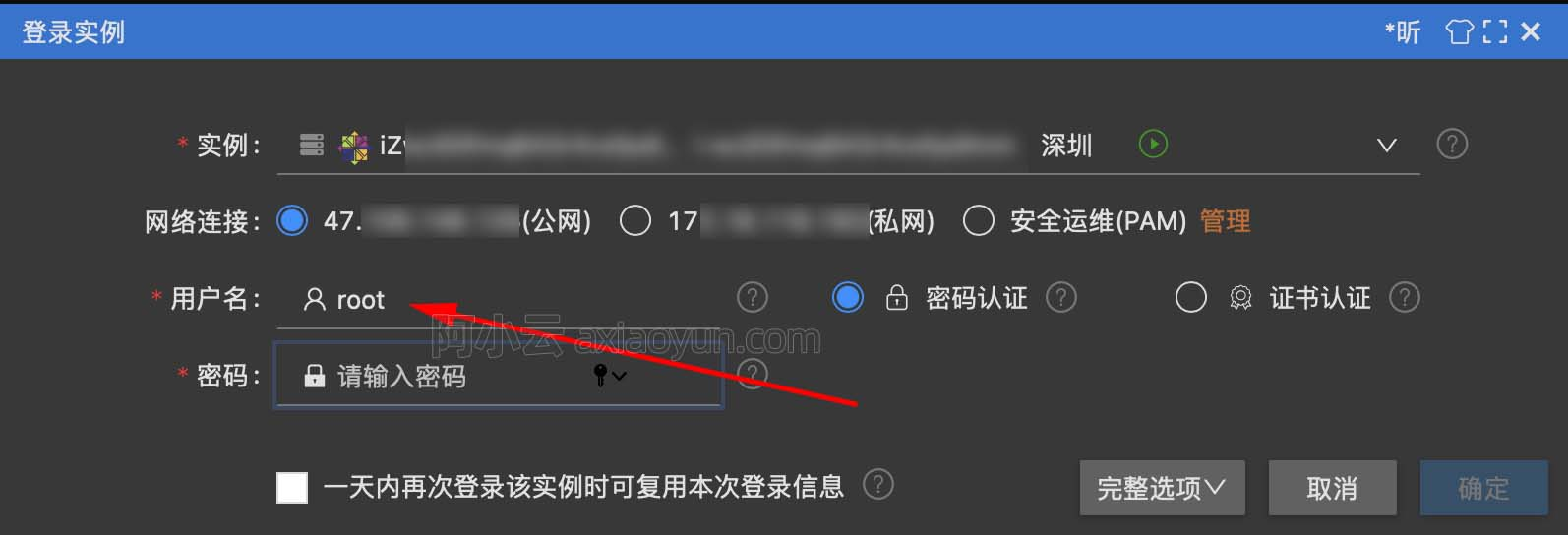+关注继续查看

正则表达式

在线正则测试工具:

http://tool.oschina.net/regex/

总结1:

1.由数字、26个英文字母或者下划线组成的字符串:
^[0-9a-zA-Z_]{1,}$2.非负整数（正整数 + 0 ）: ^/d+$
3. 正整数:
^[0-9]*[1-9][0-9]*$4.非正整数（负整数 + 0）： ^((-/d+)|(0+))$
5. 负整数 :
^-[0-9]*[1-9][0-9]*$6.整数: ^-?/d+$
7.非负浮点数（正浮点数 + 0）:
^/d+(/./d+)?$8.正浮点数 : ^(([0-9]+/.[0-9]*[1-9][0-9]*)|([0-9]*[1-9][0-9]*/.[0-9]+)|([0-9]*[1-9][0-9]*))$
9. 非正浮点数（负浮点数 + 0）:
^((-/d+(/./d+)?)|(0+(/.0+)?))$10.负浮点数 : ^(-(([0-9]+/.[0-9]*[1-9][0-9]*)|([0-9]*[1-9][0-9]*/.[0-9]+)|([0-9]*[1-9][0-9]*)))$
11. 浮点数 :
^(-?/d+)(/./d+)?$12.由26个英文字母组成的字符串 : ^[A-Za-z]+$
13. 由26个英文字母的大写组成的字符串 :
^[A-Z]+$14.由26个英文字母的小写组成的字符串 : ^[a-z]+$
15. 由数字和26个英文字母组成的字符串 :
^[A-Za-z0-9]+$16.由数字、26个英文字母或者下划线组成的字符串 : ^/w+$
17.email地址 :
^[/w-]+(/.[/w-]+)*@[/w-]+(/.[/w-]+)+$18.url: ^[a-zA-z]+://(/w+(-/w+)*)(/.(/w+(-/w+)*))*(/?/S*)?$
19. 年-月-日:
/^(d{2}|d{4})-((0([1-9]{1}))|(1[1|2]))-(([0-2]([1-9]{1}))|(3[0|1]))$/ 20.月/日/年: /^((0([1-9]{1}))|(1[1|2]))/(([0-2]([1-9]{1}))|(3[0|1]))/(d{2}|d{4})$/
21.Emil:
^([w-.]+)@(([[0-9]{1,3}.[0-9]{1,3}.[0-9]{1,3}.)|(([w-]+.)+))([a-zA-Z]{2,4}|[0-9]{1,3})(]?)$22. 电话号码: (d+-)?(d{4}-?d{7}|d{3}-?d{8}|^d{7,8})(-d+)? 23.IP地址: ^(d{1,2}|1dd|2[0-4]d|25[0-5]).(d{1,2}|1dd|2[0-4]d|25[0-5]).(d{1,2}|1dd|2[0-4]d|25[0-5]).(d{1,2}|1dd|2[0-4]d|25[0-5])$
24. 匹配中文字符的正则表达式：
[/u4e00-/u9fa5]
25.匹配双字节字符(包括汉字在内)：
[^/x00-/xff]
26. 匹配空行的正则表达式：
/n[/s| ]*/r
27.匹配HTML标记的正则表达式：
/<(.*)>.*<///1>|<(.*) //>/
28.匹配首尾空格的正则表达式：
(^/s*)|(/s*$) 29.匹配Email地址的正则表达式： /w+([-+.]/w+)*@/w+([-.]/w+)*/./w+([-.]/w+)* 30. 匹配网址URL的正则表达式： ^[a-zA-z]+://(//w+(-//w+)*)(//.(//w+(-//w+)*))*(//?//S*)?$
31. 匹配帐号是否合法(字母开头，允许5-16字节，允许字母数字下划线)：
^[a-zA-Z][a-zA-Z0-9_]{4,15}$32. 匹配国内电话号码： (/d{3}-|/d{4}-)?(/d{8}|/d{7})? 33.匹配腾讯QQ号： ^[1-9]*[1-9][0-9]*$
34. 只能输入数字：
^[0-9]*$35.只能输入n位的数字： ^/d{n}$
36.只能输入至少n位的数字：
^/d{n,}$37.只能输入m~n位的数字： ^/d{m,n}$
38.只能输入零和非零开头的数字：
^(0|[1-9][0-9]*)$39.只能输入有两位小数的正实数： ^[0-9]+(.[0-9]{2})?$
40. 只能输入有1~3位小数的正实数：
^[0-9]+(.[0-9]{1,3})?$41.只能输入非零的正整数： ^/+?[1-9][0-9]*$
42. 只能输入非零的负整数：
^/-[1-9][0-9]*$43.只能输入长度为3的字符： ^.{3}$
44. 只能输入由26个英文字母组成的字符串：
^[A-Za-z]+$45.只能输入由26个大写英文字母组成的字符串： ^[A-Z]+$
46. 只能输入由26个小写英文字母组成的字符串：
^[a-z]+$47.只能输入由数字和26个英文字母组成的字符串： ^[A-Za-z0-9]+$
48. 只能输入由数字和26个英文字母或者下划线组成的字符串：
^/w+$49.验证用户密码(正确格式为： 以字母开头，长度在5~17 之间，只能包含字符、数字和下划线) ^[a-zA-Z]/w{5,17}$
50.验证是否包含有 ^%&',;=?$/"等字符： [^%&',;=?$/x22]+
51.只能输入汉字：
^[u4e00-u9fa5]{0,}$52、只含有汉字、数字、字母、下划线不能以下划线开头和结尾 ^(?!_)(?!.*?_$)[a-zA-Z0-9_u4e00-u9fa5]+$53、只含有汉字、数字、字母、下划线，下划线位置不限 ^[a-zA-Z0-9_u4e00-u9fa5]+$
54、2~4个汉字
@"^[u4E00-u9FA5]{2,4}$总结2: 一、校验数字的表达式 1 数字：^[0-9]*$
2 n位的数字：^\d{n}$3 至少n位的数字：^\d{n,}$
4 m-n位的数字：^\d{m,n}$5 零和非零开头的数字：^(0|[1-9][0-9]*)$
6 非零开头的最多带两位小数的数字：^([1-9][0-9]*)+(.[0-9]{1,2})?$7 带1-2位小数的正数或负数：^(\-)?\d+(\.\d{1,2})?$
8 正数、负数、和小数：^(\-|\+)?\d+(\.\d+)?$9 有两位小数的正实数：^[0-9]+(.[0-9]{2})?$
10 有1~3位小数的正实数：^[0-9]+(.[0-9]{1,3})?$11 非零的正整数：^[1-9]\d*$ 或 ^([1-9][0-9]*){1,3}$或 ^\+?[1-9][0-9]*$
12 非零的负整数：^\-[1-9][]0-9"*$或 ^-[1-9]\d*$
13 非负整数：^\d+$或 ^[1-9]\d*|0$
14 非正整数：^-[1-9]\d*|0$或 ^((-\d+)|(0+))$
15 非负浮点数：^\d+(\.\d+)?$或 ^[1-9]\d*\.\d*|0\.\d*[1-9]\d*|0?\.0+|0$
16 非正浮点数：^((-\d+(\.\d+)?)|(0+(\.0+)?))$或 ^(-([1-9]\d*\.\d*|0\.\d*[1-9]\d*))|0?\.0+|0$
17 正浮点数：^[1-9]\d*\.\d*|0\.\d*[1-9]\d*$或 ^(([0-9]+\.[0-9]*[1-9][0-9]*)|([0-9]*[1-9][0-9]*\.[0-9]+)|([0-9]*[1-9][0-9]*))$
18 负浮点数：^-([1-9]\d*\.\d*|0\.\d*[1-9]\d*)$或 ^(-(([0-9]+\.[0-9]*[1-9][0-9]*)|([0-9]*[1-9][0-9]*\.[0-9]+)|([0-9]*[1-9][0-9]*)))$
19 浮点数：^(-?\d+)(\.\d+)?$或 ^-?([1-9]\d*\.\d*|0\.\d*[1-9]\d*|0?\.0+|0)$

1 汉字：^[\u4e00-\u9fa5]{0,}$2 英文和数字：^[A-Za-z0-9]+$ 或 ^[A-Za-z0-9]{4,40}$3 长度为3-20的所有字符：^.{3,20}$
4 由26个英文字母组成的字符串：^[A-Za-z]+$5 由26个大写英文字母组成的字符串：^[A-Z]+$
6 由26个小写英文字母组成的字符串：^[a-z]+$7 由数字和26个英文字母组成的字符串：^[A-Za-z0-9]+$
8 由数字、26个英文字母或者下划线组成的字符串：^\w+$或 ^\w{3,20}$
9 中文、英文、数字包括下划线：^[\u4E00-\u9FA5A-Za-z0-9_]+$10 中文、英文、数字但不包括下划线等符号：^[\u4E00-\u9FA5A-Za-z0-9]+$ 或 ^[\u4E00-\u9FA5A-Za-z0-9]{2,20}$11 可以输入含有^%&',;=?$\"等字符：[^%&',;=?$\x22]+ 12 禁止输入含有~的字符：[^~\x22]+ 三、特殊需求表达式 1 Email地址：^\w+([-+.]\w+)*@\w+([-.]\w+)*\.\w+([-.]\w+)*$
2 域名：[a-zA-Z0-9][-a-zA-Z0-9]{0,62}(/.[a-zA-Z0-9][-a-zA-Z0-9]{0,62})+/.?
3 InternetURL：[a-zA-z]+://[^\s]* 或 ^http://([\w-]+\.)+[\w-]+(/[\w-./?%&=]*)?$4 手机号码：^(13[0-9]|14[5|7]|15[0|1|2|3|5|6|7|8|9]|18[0|1|2|3|5|6|7|8|9])\d{8}$
5 电话号码("XXX-XXXXXXX"、"XXXX-XXXXXXXX"、"XXX-XXXXXXX"、"XXX-XXXXXXXX"、"XXXXXXX"和"XXXXXXXX)：^(\(\d{3,4}-)|\d{3.4}-)?\d{7,8}$6 国内电话号码(0511-4405222、021-87888822)：\d{3}-\d{8}|\d{4}-\d{7} 7 身份证号： 15或18位身份证：^\d{15}|\d{18}$
15位身份证：^[1-9]\d{7}((0\d)|(1[0-2]))(([0|1|2]\d)|3[0-1])\d{3}$18位身份证：^[1-9]\d{5}[1-9]\d{3}((0\d)|(1[0-2]))(([0|1|2]\d)|3[0-1])\d{4}$
8 短身份证号码(数字、字母x结尾)：^([0-9]){7,18}(x|X)?$或 ^\d{8,18}|[0-9x]{8,18}|[0-9X]{8,18}?$
9 帐号是否合法(字母开头，允许5-16字节，允许字母数字下划线)：^[a-zA-Z][a-zA-Z0-9_]{4,15}$10 密码(以字母开头，长度在6~18之间，只能包含字母、数字和下划线)：^[a-zA-Z]\w{5,17}$
11 强密码(必须包含大小写字母和数字的组合，不能使用特殊字符，长度在8-10之间)：^(?=.*\d)(?=.*[a-z])(?=.*[A-Z]).{8,10}$12 日期格式：^\d{4}-\d{1,2}-\d{1,2} 13 一年的12个月(01～09和1～12)：^(0?[1-9]|1[0-2])$
14 一个月的31天(01～09和1～31)：^((0?[1-9])|((1|2)[0-9])|30|31)$15 钱的输入格式： 16 1.有四种钱的表示形式我们可以接受:"10000.00" 和 "10,000.00", 和没有 "分" 的 "10000" 和 "10,000"：^[1-9][0-9]*$
17 2.这表示任意一个不以0开头的数字,但是,这也意味着一个字符"0"不通过,所以我们采用下面的形式：^(0|[1-9][0-9]*)$18 3.一个0或者一个不以0开头的数字.我们还可以允许开头有一个负号：^(0|-?[1-9][0-9]*)$
19 4.这表示一个0或者一个可能为负的开头不为0的数字.让用户以0开头好了.把负号的也去掉,因为钱总不能是负的吧.下面我们要加的是说明可能的小数部分：^[0-9]+(.[0-9]+)?$20 5.必须说明的是,小数点后面至少应该有1位数,所以"10."是不通过的,但是 "10" 和 "10.2" 是通过的：^[0-9]+(.[0-9]{2})?$
21 6.这样我们规定小数点后面必须有两位,如果你认为太苛刻了,可以这样：^[0-9]+(.[0-9]{1,2})?$22 7.这样就允许用户只写一位小数.下面我们该考虑数字中的逗号了,我们可以这样：^[0-9]{1,3}(,[0-9]{3})*(.[0-9]{1,2})?$
23 8.1到3个数字,后面跟着任意个 逗号+3个数字,逗号成为可选,而不是必须：^([0-9]+|[0-9]{1,3}(,[0-9]{3})*)(.[0-9]{1,2})?$24 备注：这就是最终结果了,别忘了"+"可以用"*"替代如果你觉得空字符串也可以接受的话(奇怪,为什么?)最后,别忘了在用函数时去掉去掉那个反斜杠,一般的错误都在这里 25 xml文件：^([a-zA-Z]+-?)+[a-zA-Z0-9]+\\.[x|X][m|M][l|L]$
26 中文字符的正则表达式：[\u4e00-\u9fa5]
27 双字节字符：[^\x00-\xff] (包括汉字在内，可以用来计算字符串的长度(一个双字节字符长度计2，ASCII字符计1))
28 空白行的正则表达式：\n\s*\r (可以用来删除空白行)
29 HTML标记的正则表达式：<(\S*?)[^>]*>.*?</\1>|<.*? /> (网上流传的版本太糟糕，上面这个也仅仅能部分，对于复杂的嵌套标记依旧无能为力)
30 首尾空白字符的正则表达式：^\s*|\s*$或(^\s*)|(\s*$) (可以用来删除行首行尾的空白字符(包括空格、制表符、换页符等等)，非常有用的表达式)
31 腾讯QQ号：[1-9][0-9]{4,} (腾讯QQ号从10000开始)
32 中国邮政编码：[1-9]\d{5}(?!\d) (中国邮政编码为6位数字)
33 IP地址：\d+\.\d+\.\d+\.\d+ (提取IP地址时有用)10099 026799 06767 014503 011643 013896 0windows server 2008阿里云ECS服务器安全设置

9161 07366 04511 054770 0
76

0

《2021云上架构与运维峰会演讲合集》

《零基础CSS入门教程》

《零基础HTML入门教程》